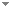Switch to: Citations

Programming Planck units from a virtual electron; a Simulation Hypothesis (summary)

Eur. Phys. J. Plus 133:278 (2018)

 .Nick Bostrom & Julian Savulescu - 2009 - Oxford University Press.No categories  Export citationBookmark76 citations Programming Planck Units From a Virtual Electron; a Simulation Hypothesis.Malcolm J. Macleod - 2018 - European Physical Journal Plus 133:278.The simulation hypothesis proposes that all of reality is an artificial simulation. In this article I describe a simulation model that derives Planck level units as geometrical forms from a virtual (dimensionless) electron formula $f_e$ that is constructed from 2 unit-less mathematical constants; the fine structure constant $\alpha$ and $\Omega$ = 2.00713494... ($f_e = 4\pi^2r^3, r = 2^6 3 \pi^2 \alpha \Omega^5$). The mass, space, time, charge units are embedded in $f_e$ according to these ratio; ${M^9T^{11}/L^{15}} = (AL)^3/T$ (units = (...) Direct download     Export citationBookmark5 citations The Mathematical Universe.Max Tegmark - 2007 - Foundations of Physics 38 (2):101-150.I explore physics implications of the External Reality Hypothesis (ERH) that there exists an external physical reality completely independent of us humans. I argue that with a sufficiently broad definition of mathematics, it implies the Mathematical Universe Hypothesis (MUH) that our physical world is an abstract mathematical structure. I discuss various implications of the ERH and MUH, ranging from standard physics topics like symmetries, irreducible representations, units, free parameters, randomness and initial conditions to broader issues like consciousness, parallel universes and (...) Direct download (4 more)     Export citationBookmark88 citations